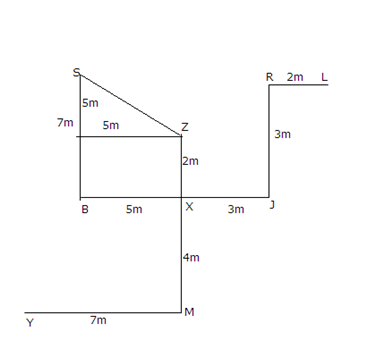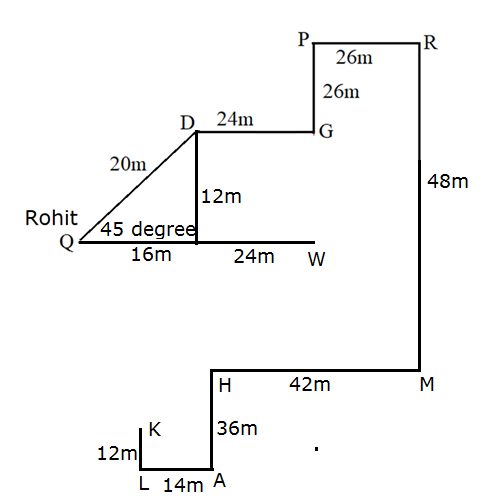# SBI Clerk Mains Reasoning (Day-91)

Dear Aspirants, Our IBPS Guide team is providing new series of Reasoning Questions for SBI Clerk Mains 2020 so the aspirants can practice it on a daily basis. These questions are framed by our skilled experts after understanding your needs thoroughly. Aspirants can practice these new series questions daily to familiarize with the exact exam pattern and make your preparation effective.

Start Quiz

Ensure Your Ability Before the ExamTake SBI Clerk 2020 Mains Free Mock Test

Direction Sense

Direction (1-3): Study the following information carefully and answer the questions given below:

Point X is midpoint for Point B and Point J as well as for Point Z and Point M. Points Z and M starts from north to south respectively. Points B and J is from West to East respectively. Point R is 3m north of Point J. Point L is 2m east of Point R. Distance between points X and Z is 1m less than distance between points R and J. Distance between points X and M is twice of X and Z. Distance between Points X and B is more than X and J. Point M is 7m east of Point Y. Lines XM and Point BX are in the distance of consecutive number.Also X&M and X&J are in consecutive distance.

1) Which of the following statement is true?

A) Point L is south west of Point X

B) If Point Z is 5m west of Point V, then Point V is 1m north of Point L

C) Points RLZ forms a straight line

D) Point R is east of Point Z

E) None is true

2) If Point Y is 6m south of Point H, then How far and in which direction Point H with respect to Point Z?

A) 6m, West

B) 5m, East

C) 7m, West

D) 3m, East

E) None of these

3) If Point B is south of Point S, Distance between Point YM is same as the distance between Point BS, What is the distance between Point Z and Point S?

A) 5m

B) 5 √2 m

C) 3 √5

D) 6m

E) None of these

Directions (4-6): Study the following information carefully and answer the questions given below it.

Two personsRohit and Mohit standing at point Q and point K respectively. Point D is 20m north-east of point Q which is exactly 450. Point K is 12m north of point L, which is 14m west of point A. Point P is 26m west of point R and is 26m north of point G. Point W is 40m east of point Q. point M is 42m east of point H and is 48m south of point R. Point G is 24m east of point D and is north of point W. point H is 36m north of point A.

4) What is the position of Mohit with respect to Point G?

A) South-West

B) South

C) North-East

D) North

E) None of the above

5) What is the distance between point W and point P?

A) 54m

B) 38m

C) 64m

D) 52m

E) Cannot be determined

6) What is the position of point D with respect to point W?

A) North

B) West

C) South-East

D) North-West

E) None of the above

Logical reasoning

Directions (7): Read the situation given below and choose the correct answer out of the 5 options.

Kisanlal is a farmer in Sanjarpur, a village in UP. He owns a small plot of land on which he grows sugarcane. Kisanlal is facing some troubles in farming, he has the seeds, the pesticides, fertilizers but he doesn’t have a tractor or any other types of equipment to plough the field. Rajpal, another village farmer has a tractor and the ploughing equipment but cannot afford fertilizers or pesticides for his crops. And most of the farmers in the village face the same problem.

7) What should the farmers do?

A) They should take loans from money lenders.

B) Kisanlal should steal Rajpal’s resources.

C) Rajpal should not use fertilizers and pesticides and let the crops be of poor quality.

D) All the farmers should start a cooperative.

E) The farmers should sell off their lands and migrate to the city

Inequality

Directions (8-10): In each of the following questions, relationship between different elements is shown in the statement followed by three sets of conclusion. Find the conclusion which is definitely true.

8) Statement:

R ≥ H > T < M; H > W = U < Q; U = B > F = S

Conclusion:

I). Q > T

II). R ≥ B

III). S < Q

A) Only I follows

B) Only II and III follows

C) Only I and III follows

D) Only III follows

E) None follows

9) Statement:

W < Z = Y; D > H ≤ W < U; H ≥ B = T < Q; B > S ≤ F

Conclusion:

I). D > S

II). Y ≥ T

III). T < U

A) Only I follows

B) Only II and III follows

C) Only I and III follows

D)  Only III follows

E) None follows

10) Statement:

M < G = E; U = Q > B; K = M < U ≤ D; Q < F = R

Conclusion:

I). E > B

II). K = F

III). R > K

A) Only III follows

B) Either II or III follows

C) Only I and II or III follows

D) Only I and III follows

E) None follows

Directions (1-3):Directions (4-6):As all the farmers are lacking something or the other, so if they come together as a cooperative they can pool their resources and use them with no additional costs and hence cultivate efficiently. While all other options make them pay from their pockets or guarantee uncertainties as in the case of migrating to the city. Option 4 is the only one providing an alternative which is helping everyone out and also ensuring that resources don’t go to waste.

Directions (8-10):